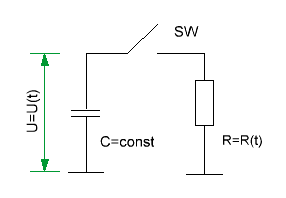Research website of Vyacheslav Gorchilin
2016-07-29
Parametric variation of the resistance, capacitance and inductance in RLC-circuits
1. Parametric variation of the resistance
Consider a simple RC-chain, but with one addition — the resistance $$R$$ here parametrically varies with time: $$R = R(t)$$. Capacity $$C$$ are constant in time, but is pre-charged to a voltage $$U_{0}$$.I.e. at the initial moment of time, before the circuit key SW: $U(0)=U_{0} \qquad (1.1)$
Make the equation for this circuit assuming that the key SW is closed at time $$t=0$$: ${dU(t) \over dt} + {U(t) \over C \ R(t)} = 0 \qquad (1.2)$ we have Before us a homogeneous differential equation of the first order. After his decision  and finding of the initial coefficients, we obtain the final dependence of the voltage on the capacitor from time to time: $U(t) = U_{0} \ exp \left( -{1 \over C} \int {dt \over R(t)} \right) \qquad (1.3)$
But we need to get the value of energy that will get resistance by discharge of the capacitor. To do this, recall the school formula for power is: $I(t) = {dQ(t) \over dt}$ where $$Q$$ is the charge located in the condenser. Also recall that: $$Q(t) = C\,U(t)$$. To find the total energy $$W_{R}$$ that passes through $$R$$, we apply the well-known formula in integral form: $W_{R} = \int_0^\tau I(t)^{2} \, R(t) \, dt \qquad (1.4)$ where $$\tau$$ — discharge time. Substituting all the previously obtained formulas, differentiating and making the substitution, we obtain the final result: $W_{R} = { C\,U_{0}^2 \over 2 } \left( 1 - exp \left( -{2 \over C} \int_0^\tau {dt \over R(t)} \right) \right) \qquad (1.5)$
Now we have to find the energy $$W_{c0}$$, which we had to pre-charge the capacitor, subtract from it remaining in the case, if we don't expect full charge, and then compare the expended energy $$W_{C}$$ and stress $$W_{R}$$.
The total potential energy of the capacitor is on the well-known formula: $W_{c0} = {C\,U_{0}^2 \over 2},$ but if you don't wait for the full discharge of the capacitor and open circuit key SW at the time $$\tau$$, then the remaining energy will be so: $W_{c\tau} = {C\,U(\tau)^2 \over 2} \qquad (1.6)$ the voltage on the capacitor, when opening the key, is given by (1.3): $U(\tau) = U_{0} \ exp \bigg( -{1 \over C} \int_0^\tau {dt \over R(t)} \bigg) \qquad (1.7)$ Thus, the spent energy will be, as the difference $$W_{c0}$$ and $$W_{c\tau}$$: $W_{C} = W_{c0} - W_{c\tau} = { C\,U_{0}^2 \over 2 } \left( 1 - exp \left( -{2 \over C} \int_0^\tau {dt \over R(t)} \right) \right) \qquad (1.8)$ Comparing formulas (1.5) and (1.8) we see that $$W_{C}$$ and $$W_{R}$$ equal, and it does not depend either on the properties of parametric resistance, or when opening a key.

Thus, in an RC circuit with a constant capacitance it is impossible to gain energy, even if the resistance is parametric!

The proof for RL-circuit is similar, only instead of voltage is substituted for current $$I$$ instead of $$C$$ is the inductance $$L$$ and $$R$$ is moved from the denominator to the numerator: ${dI(t) \over dt} + {I(t) \, R(t) \over L} = 0$ Is a proof the reader can infer on their own, but it also shows that the case of constant inductance, RL-circuits also cannot be receiving increase energy, even if $$R$$ — dimensional.